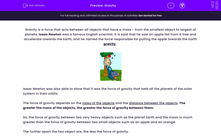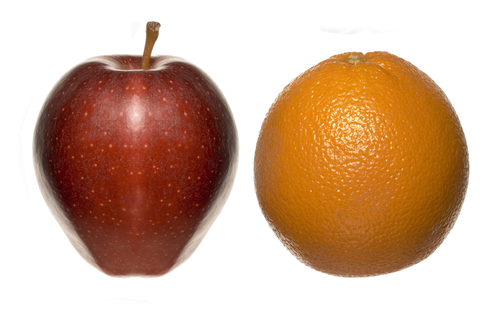# Relate Gravity and Weight

In this worksheet, students will explore the force that keeps our feet on the ground and stops the moon or satellites from moving off into space: gravity.Key stage:  KS 3

Curriculum topic:   Physics: Motion and Forces

Curriculum subtopic:   Forces

Popular topics:   Physics worksheets

Difficulty level:#### Worksheet Overview

Gravity is a force that acts between all objects that have a mass - from the smallest object to the largest of planets. Isaac Newton, the famous English scientist, said that he saw an apple fall from a tree and accelerate towards the Earth, and he named the force responsible for pulling the apple towards the Earth gravityIsaac Newton was also able to show that it was the force of gravity that held all the planets of the Solar System in their orbits.

The force of gravity depends on the mass of the objects and the distance between the objects. The greater the mass of the objects, the greater the force of gravity between them.

So, the force of gravity between two very heavy objects such as the planet Earth and the moon is much greater than the force of gravity between two small objects such as an apple and an orange.

The further apart the two objects are, the less the force of gravity.It is a large force of gravity that holds the moon in orbit around the Earth. The  gravitational force of the moon is responsible for the tides.Only a very weak force of gravity would occur between two objects of low mass. The force is so weak that we are unable to feel it.

The gravitational force between an object and the Earth is known as the weight of the object. On Earth, the force of gravity pulls objects down towards the centre of the Earth and the weight of the object can be calculated using the gravitational field strength.

Gravitational field strength on the Earth is equal to 10 N/kg (Newtons per kilogram).

### What is EdPlace?

We're your National Curriculum aligned online education content provider helping each child succeed in English, maths and science from year 1 to GCSE. With an EdPlace account you’ll be able to track and measure progress, helping each child achieve their best. We build confidence and attainment by personalising each child’s learning at a level that suits them.

Get started